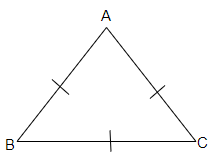# Show that the angles of an equilateral triangle are $60^{\circ}$ each.

To do:

We have to show that the angles of an equilateral triangle are $60^o$ each.

Solution:Let us consider an equilateral triangle $ABC$

We have,

$AB=BC=AC$ (from fig)

We know that

The sides opposite to the equal angles are equal.

Therefore,

$\angle A=\angle B=\angle C$

We also know that,

The sum of the interior angles of a triangle is always equal to $180^o$

This implies

In $\triangle ABC,$

$\angle A+\angle B+\angle C = 180^o$

Therefore,

$3\angle A=180^o$  (since $\angle A=\angle B=\angle C$)

$\angle A=\frac{180^o}{3}$

$\angle A= 60^o$

Therefore,

$\angle A=\angle B=\angle C=60^o$

Hence the angles of an equilateral triangle are $60^o$ each.

Updated on: 10-Oct-2022

30 Views